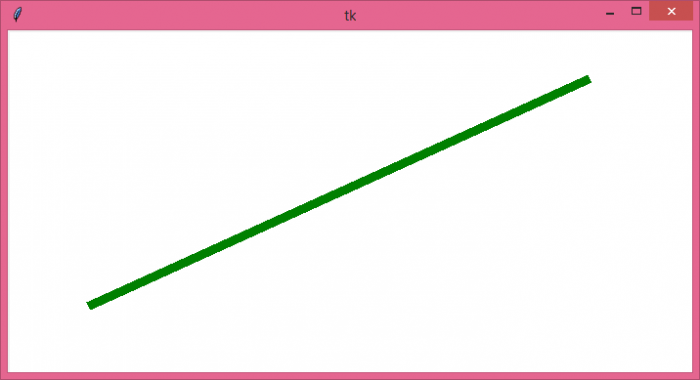# Drawing a line between two mouse clicks using tkinter

TkinterPythonGUI-Programming

Consider a case for creating a GUI application such that when we click on the window with a mouse button, it stores the coordinates and creates a line between two given points. Tkinter provides events that allow the user to bind the keys or buttons with the functions.

To draw a line between two points, we can follow these general steps,

• Create a canvas widget and pack it to display in the window.

• Define a function draw_line() that works as the event when the user does the click event.

• Create a global variable that counts the number of clicks in the canvas.

• If the count becomes two, then draw a line between the first and second coordinates.

• Bind the Mouse button with the callback function to get full control over the function.

## Example

# Import the required libraries
from tkinter import *
from tkinter import ttk

# Create an instance of tkinter frame or window
win=Tk()

# Set the size of the window
win.geometry("700x350")

# Define a function to draw the line between two points
def draw_line(event):
global click_num
global x1,y1
if click_num==0:
x1=event.x
y1=event.y
click_num=1
else:
x2=event.x
y2=event.y
# Draw the line in the given co-ordinates
canvas.create_line(x1,y1,x2,y2, fill="green", width=10)

# Create a canvas widget
canvas=Canvas(win, width=700, height=350, background="white")
canvas.grid(row=0, column=0)
canvas.bind('<Button-1>', draw_line)
click_num=0

win.mainloop()

## Output

Run the above code to display a window. If we click on the canvas widget twice anywhere, it will draw a line in the canvas.